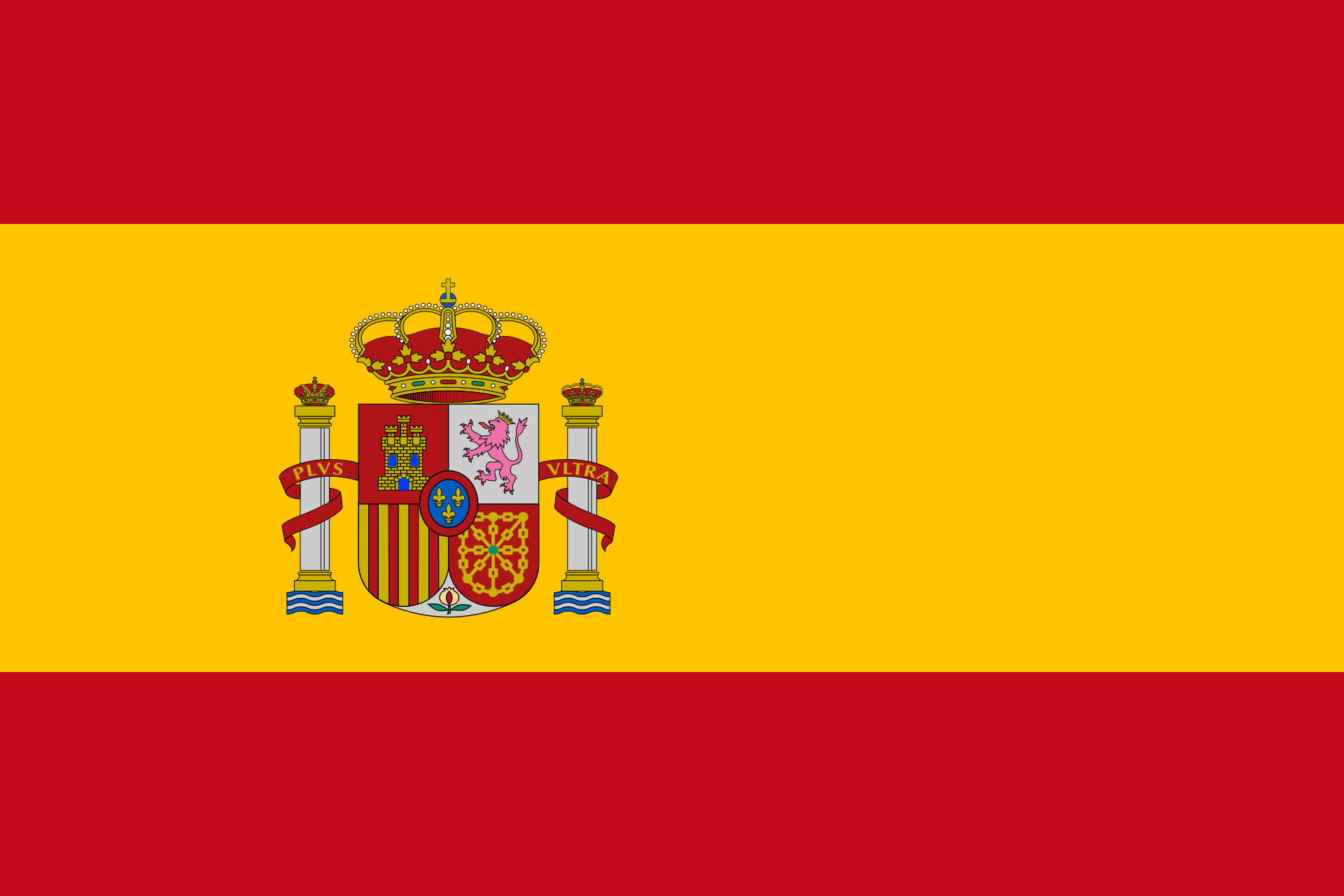# Cross productEnter two vectors. Mathepower calculates their cross product.

 u =and v=## What is the use of the cross product?

The cross product is a good way to find a vector staning perpendicular on two other vectors.

## How to calculate the cross product?

It is not too easily explained, and furthermore, there is a change of signs mistake danger. You take (maybe hence the name) always the over-cross-product of two components. This means: First component of first vector times second component of second vector. Then, you calculate first component of second vector times second component of first vector. Calculate the difference of those products and write it into the third line of the cross productģ Generally, in every line you find the result of cross multiplying the other numbers (but in the second line with inverted sign).

## This sounds confusing. How to do it?

Just enter your numbers above and click on ""Calculate"". This is better understood by taking a look at an example, for sure.